## Understanding The Order of Checked Boxes In Tableau's Table Calculations - Tableau Tips With TableauTimothy

by Timothy Manning

When doing a table calculation, Tableau uses a system of ‘checked’ and ‘unchecked’ boxes to determine how fields should be used in the calculation.

For example, if our fields are region, segment and sales, are we calculating the percent of total sales for each region per segment?

Or are we calculating the percent of total sales for each segment per region?

Check out Joe Macari’s (of Data School Cohort 7) blog to understand the difference between a ‘checked’ and ‘unchecked’ box, and how to read it in plain English.

What I wish to talk about however, concerns changing the order of the ‘checked’ boxes (in the table calculation window). You may have thought that the order doesn’t matter, but hopefully after this blog post, you’ll understand how it changes the way Tableau computes the table calculation.

Let’s take a look at an example using the Tableau default data set, Superstore.

# 1. Creating The View

With year and quarter on rows, category on columns, and SUM(Sales) in the table: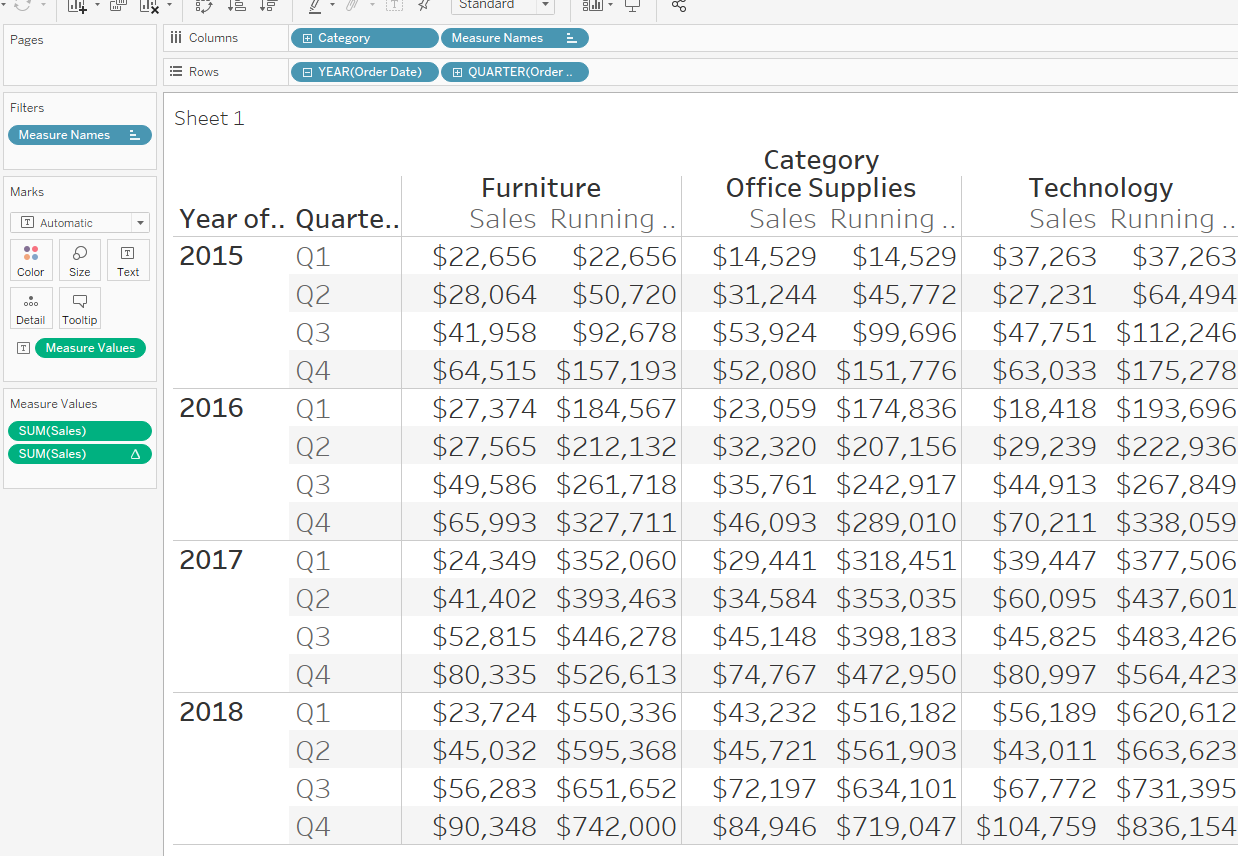Add a running total table calculation that should look like this: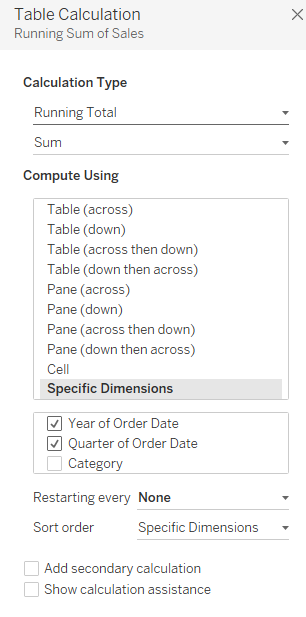The term ‘order of the checked boxes’ refers to Year of Order Date being ordered above or below Quarter of Order Date in the calculation window.

# 2. Understanding The Initial Table Calculation

To understand how this running total is being computed by Tableau, we look at the ‘checked’ and ‘unchecked’ boxes:

• Category is ‘unchecked’ so the running total starts again for each category
• Year and Quarter are ‘checked’ (and year is placed above quarter in the calculation) so the sales are cumulatively added up by year and then by quarter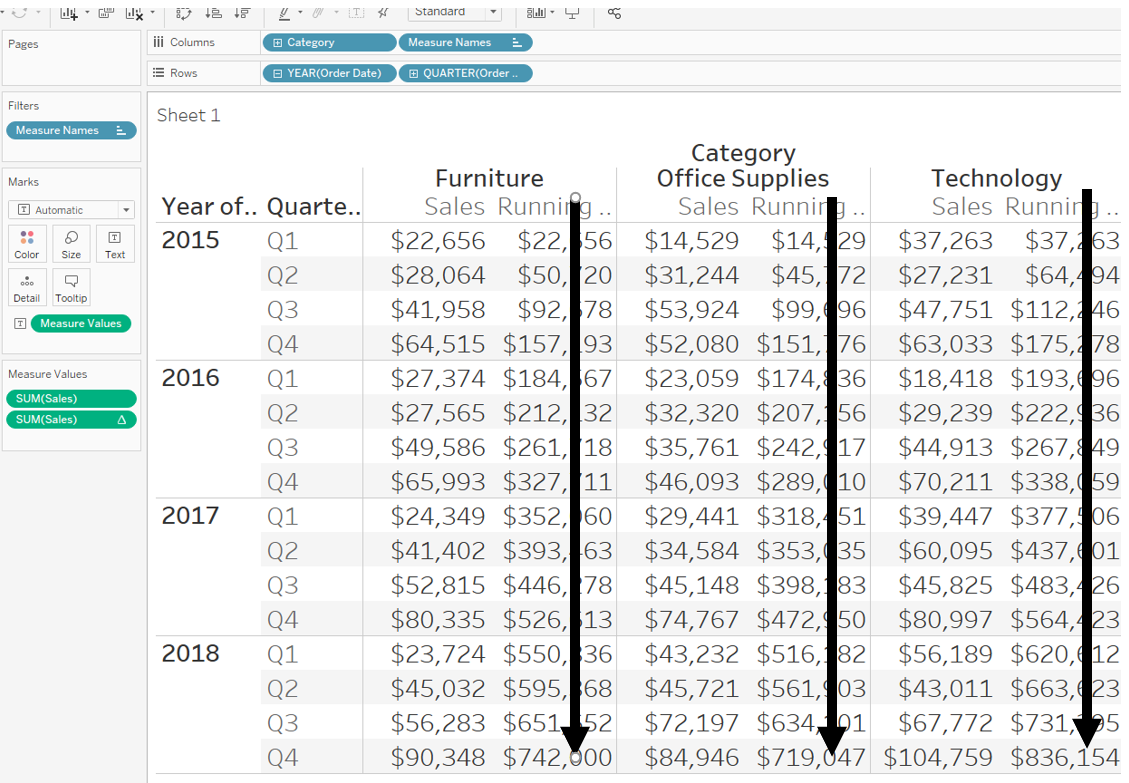so we start at Q1 of 2015, then add the sales from Q2 of 2015, then Q3 of 2015, then Q4 of 2015, then Q1 of 2016, then Q2 of 2016 etc.

# 3. What Happens If We Change The Order Of The ‘Checked’ Boxes?

If we swap year and quarter in the table calculation (by dragging one above or below the other) to look like this: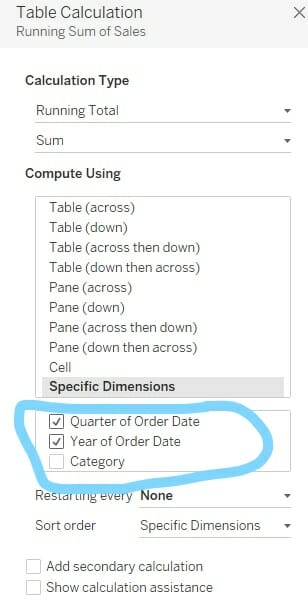then the sales are cumulatively added by quarter first and then by year, giving this table: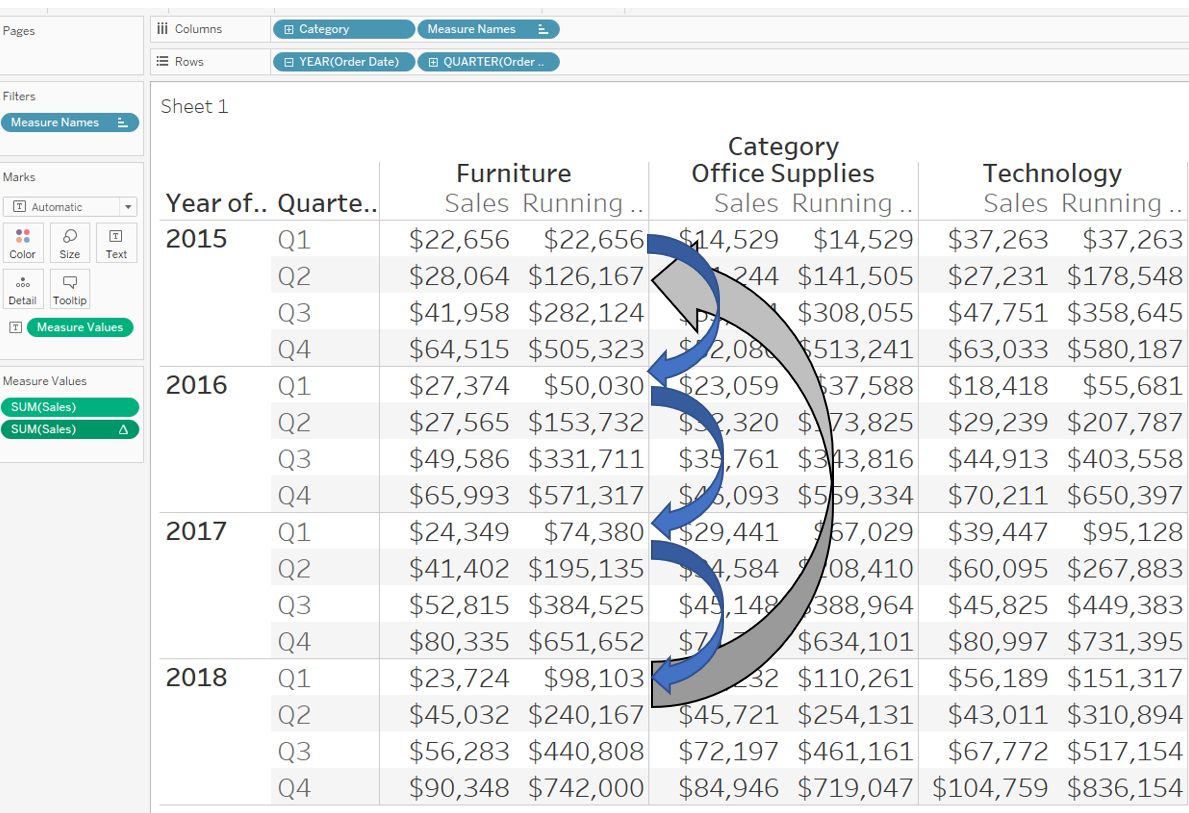so now we start at Q1 of 2015, then add the sales from Q1 of 2016, then Q1 of 2017, then Q1 of 2018, then Q2 of 2015, then Q2 of 2016 etc.

This calculation doesn’t make sense for the table we have currently, so let’s change the order of the dimensions on rows, putting quarter first. Now the running total runs down the table which makes more sense.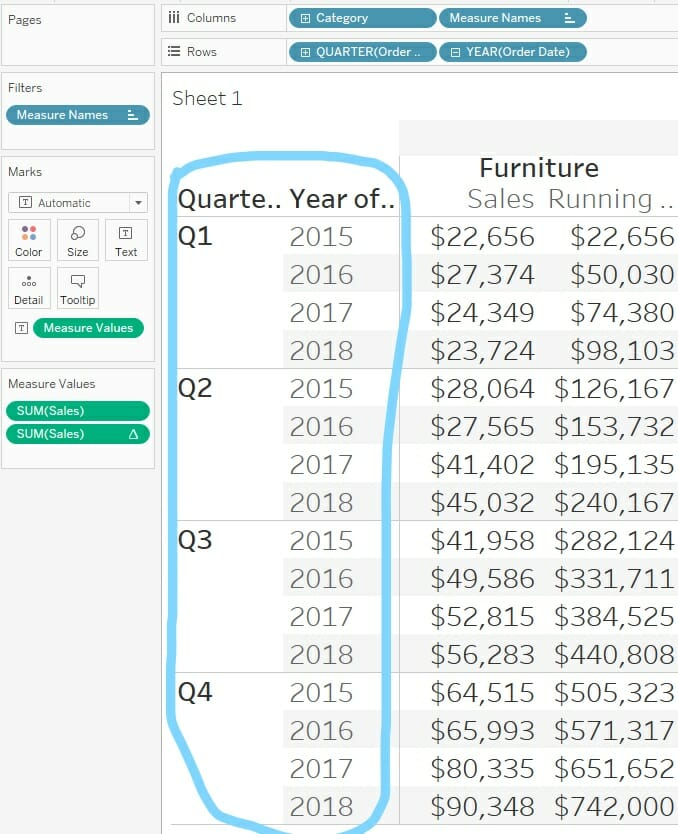# 4. Summary

In summary, the order of the ‘checked’ boxes does therefore change the way Tableau computes the table calculation!

I always find it really helps to build out the table like this before making the chart, to check my calculations are set up correctly.

I hope you enjoyed this blog! For more from me, check out my Twitter and LinkedIn pages.Timothy Manning

Sun 10 Jun 2018

Sat 28 Apr 2018

Fri 27 Apr 2018

Thu 26 Apr 2018